×The Free 30-Day CAT RC Course

"It is designed to help you excel in the upcoming CAT 2023 exam"

-By Lavleen Kaur Kapoor. Over 1,73,000 Subscribers

No thanks >

-->

1.    Three tangents of a circle with centre O from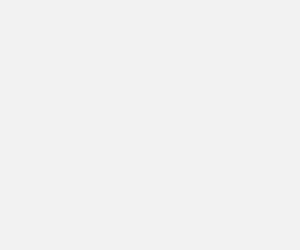APB. If o, findA. 60o
B. 110o
C. 75o
D. 140o
E. None of these

2. A fly is trapped inside a hollow cube. It moves from A to B along the edges of the cube, taking the shortest possible route. It then comes back to A, again along the edges, taking the longest route (without going over any point more than once). If the total distance travelled is 5,040 m, what is the area of a face of the cube?A. 99,225 m2
B. 32,400 m2
C. 3,96,900 m2
D. 46,225 m2
E. None of these

3. In the given parallelogram ABCD, if 3(BE) = 2(DC) and the area ofDQC is 36, find out the area ofBQE.A. 16
B. 20
C. 24
D. 18
E. None of these

4. In the given figure, BO and CO are the bisectors ofA. 75o
B. 65o
C. 90o
D. 80o
E. None of these

5.  In the following figure, if PQ = R(P is the centre of the bigger circle) and the shaded area is equal to A, then the radius of the inner circle is equal to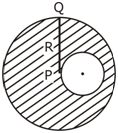A.B.C.D.6.  Find the value of x in the following figure.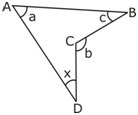A. 360o – (a + b + c)
B. b – a – c
C. 180o – c
D. 180o + c
E. None of these

7. If O is the centre of the circle then find the value of (x + y).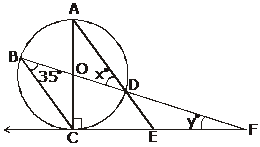A. 35
B. 20
C. 70
D. 90
E. None of these

8. If C is the center of two concentric circles as shown in the figure and mo, then find m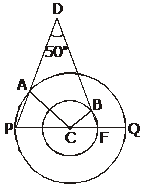A. 50o
B. 80o
C. 100o
D. 130o
E. 135o

9. In the given figure if B is the midpoint of AC then find m + n +5/2.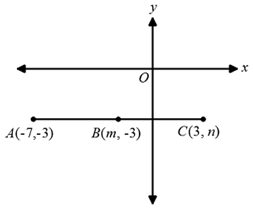A. 0
B. 1
C. 2
D. -1
E. -2

10. If A (–2, –1), B(a, 0), C(4, b) and D(1, 2) are the vertices of a parallelogram, find the values of a + b.

A. 3
B. 4
C. 5
D. 6
E. 8

## Solution

1. B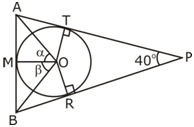It is clear that oAT = AM and BR = BM
(Length of the tangents from an external point)
Hence, angle at the centre of the circle must be equal.
So We have to find= 360 – 140

[o, calculated earlier]= 220/2 = 1100

2. C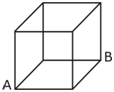As cube shown in the figure where to reach from A to B by the shortest route will be 2x (if x be the length of the side of the cube), and the longest route from B to A will be 6x.

Hence, 2x + 6x = 5040;  x = 5040/8 = 360mArea of a face = x2 = (630)2 = 396900 m2

3.  A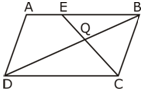It is given that 3BE = 2DC or BE/DC = 2/3

SinceDCQ andBQE are similar,or Ar(BQE) = 36 x 4/9 = 16

4.    B5. D

Here as given that PQ = R

Let us assume r be the radius of the inner circle.6. B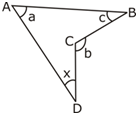Interior angle DCB = 3600 –b. Now, in quadrilateral ABCD, a + x + 3600 –b +c = 3600 => x = b – a - c.

7. E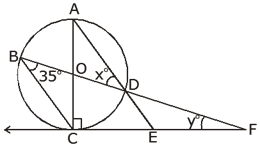Angles drawn on the same arc and in the same segment are equal).0 . Now inBCF , 350 + (x+900) + y = 1800

=> x + y =1800 - 1250 = 550.

8. B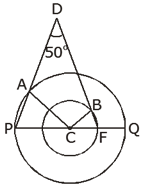9. ASimilarly,10. B

We know that the diagonals of a parallelogram bisect each other. So, the coordinates of the mid-point of AC are same as the coordinates of the mid-point of BD.